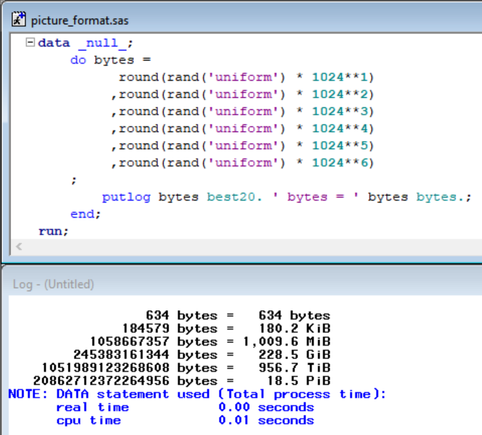## Juletip #8 - How many kilobytes in 5,000 bytes?

5 obviously since 5000 / 1000 = 5. But how many kibibytes then?

https://en.wikipedia.org/wiki/Kibibyte

This handy SAS picture format will tell you! 4.8 kibibytes (KiB)

``````proc format;
picture bytes
0    - 1023    = '0,009 bytes'
1024 - 1048575 = '0,000.0 KiB' (mult = 0.009765625)
;
run;``````

The first range from 0 to 1023 is formatted as bytes. A value in this range is being put into the template '0,009 bytes' which indicates that we want to print digits (0) with a thousand separator (,) and that we want to print zero values (9).

The next range is formatted as KiB and goes from 1024 to 1048575 since 1024 * 1024 - 1 = 1048575. To fit values in this range into the template we first need to divide the value by 1024 to get the number of KiB. Then we need to multiply it by 10 to fit in one decimal. The multiplier option can do the calculation like this 1 / 1024 * 10 = 0.009765625

But we don't have to do any calculations before defining the format. Using %EVAL and %SYSEVALF the values will be calculated when the format is being compiled.

``````proc format;
picture bytes
0              - %eval(1024**1 - 1) = '0,009 bytes'
%eval(1024**1) - %eval(1024**2 - 1) = '0,000.0 KiB' (mult = %sysevalf(1 / 1024**1 * 10))
;
run;``````

This will make the code more readable because we expose the logic behind it. Also, it will make it very easy to expand it.

``````proc format;
picture bytes
0              - %eval(1024**1 - 1) = '0,009 bytes'
%eval(1024**1) - %eval(1024**2 - 1) = '0,000.0 KiB' (mult = %sysevalf(1 / 1024**1 * 10))
%eval(1024**2) - %eval(1024**3 - 1) = '0,000.0 MiB' (mult = %sysevalf(1 / 1024**2 * 10))
%eval(1024**3) - %eval(1024**4 - 1) = '0,000.0 GiB' (mult = %sysevalf(1 / 1024**3 * 10))
%eval(1024**4) - %eval(1024**5 - 1) = '0,000.0 TiB' (mult = %sysevalf(1 / 1024**4 * 10))
%eval(1024**5) - high               = '0,000.0 PiB' (mult = %sysevalf(1 / 1024**5 * 10))
other                               = ' '
;
run;``````

Running the format on some random bytes and it looks like this:For a more detailed explanation of picture formats have a look at this paper http://www2.sas.com/proceedings/sugi31/243-31.pdf or the SAS documentation https://go.documentation.sas.com/?docsetId=proc&docsetTarget=p0n990vq8gxca6n1vnsracr6jp2c.htm&docset...

## Re: Juletip #8 - How many kilobytes in 5,000 bytes?

Very cool 🙂 Expands the already great SIZEKMG Format and illustrates the use of picture formats very well.

This definitely goes into my catalog of user defined formats.

Discussion stats